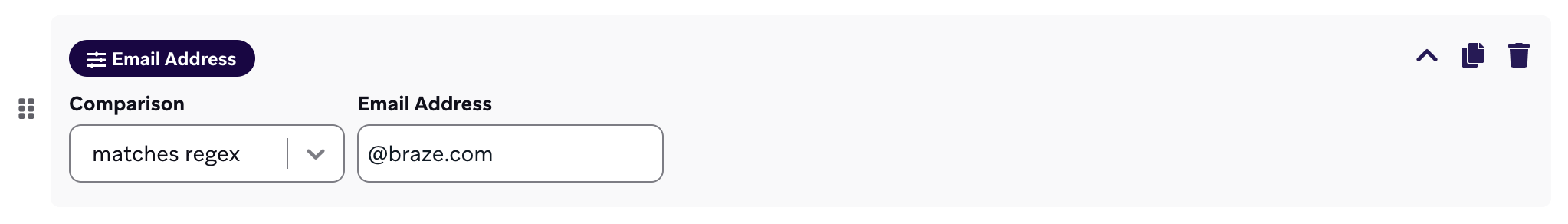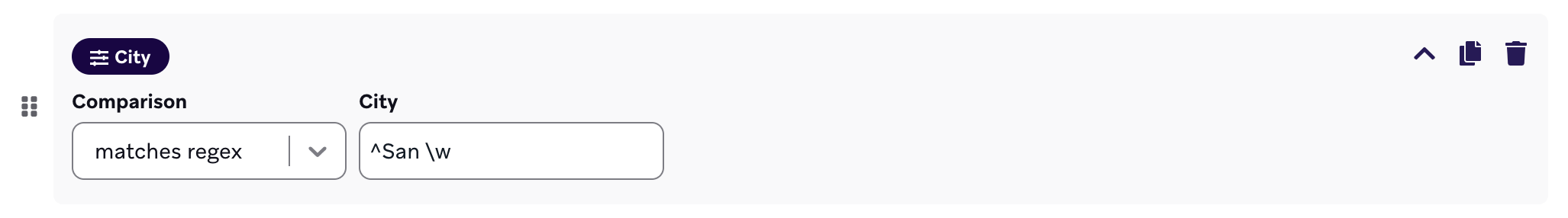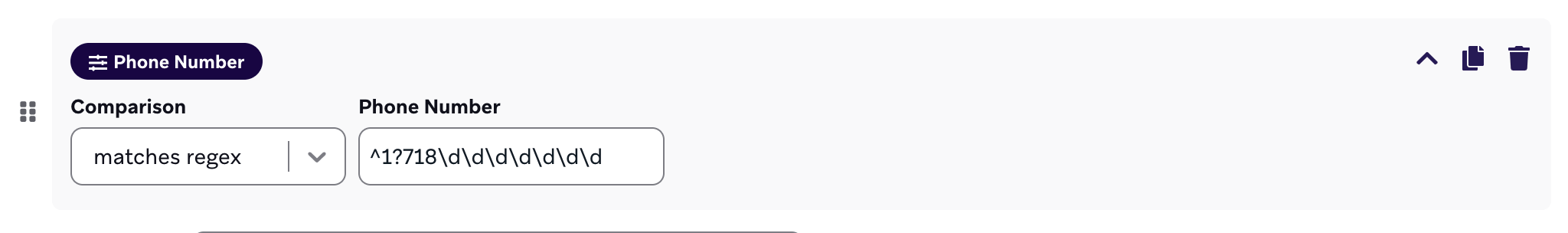#Regular expressions with Braze

This reference article covers what regular expressions are, how to begin using them, and offers debugger functionality to validate and test regular expressions.

A regular expression, known commonly as a regex, is a sequence of characters that defines a search pattern. Regular expressions let you validate text groupings and perform find and replace actions. At Braze, we leverage regular expressions to give you a more flexible string matching solution in your segmentation and campaign filtering for your target audience.

In the linked Braze Learning course, we show you how regular expressions can be used and tested on Regex101. We also offer an inhouse regex tester, a helpful cheatsheet, sample data referenced in the regex Braze Learning video, as well as some frequently asked questions.

## Regex debugger

This form allows for basic validation and testing of regular expressions. ​

Regex: ​
/
/gi

Check Value(s):

​ Matched Results:

#### How do I filter for inbox-specific email addresses when segmenting?

Use the email address filter, set it to `matches regex`. Then reference the regex for email addresses:

``````1
[a-zA-Z0-9.+_-]+@[a-zA-Z0-9.-]+\.[a-zA-Z.-]+
``````

We can break this regex down to the following three parts:

• `[a-zA-Z0-9.+_-]+` is the beginning of the email address before the at `@` character. So the “name” in “name@example.com”.
• `[a-zA-Z0-9.-]+` is the first part of the domain. So the “example” in “name@example.com”.
• `[a-zA-Z.-]+` is the last part of the domain. So the “com” in “name@example.com”.

#### How do I filter for email addresses associated to a specific domain?

Say you want to filter for emails ending with “@braze.com”. You would use the email address filter, set it to `matches regex`, and enter “@braze.com” in the regex field. The same applies for any other email domain.#### How can I use filter number strings for values ≥ x or ≤ x?

If you’re searching for values greater than or equal to (≥) x, use the following regex:

``````1
^([x-y]|\d{z,})\$
``````

Where `x-y` is the range of numbers (0-9) of the first digit, and `z` is the one more the number of digits of x. For example, for values greater than or equal to 50, the regex would then be `^([5-9][0-9]|\d{3,})\$`.

If you’re searching for values less than or equal to (≤) x, use the following regex:

``````1
^([x-y]|[a-b])\$
``````

Where `x-y` is the range of numbers (0-9) of the first digit, and `a-b` is the lower bound range of x. For example, for values less than or equal to 50, the regex would then be `^([5-9][0-9]|[0-4][0-9])\$`.

#### How do I filter custom attributes that start with a specific string?

Use the caret symbol (`^`) to denote what the string starts with, then enter the name of the custom attribute you want to specify.

For example, if you’re trying to target users who live in cities that start with “San”, your regex would be `^San \w`. With this regex, you would successfully target users from cities like San Francisco, San Diego, San Jose, and so on.#### How do I filter for specific phone numbers?

Before using regex to filter phone numbers, remember that numbers logged for user profiles must be in E.164 format, as specified in User phone numbers.

Assuming you’re searching for US phone numbers, use the regex format `1?\d\d\d\d\d\d\d\d\d\d`, where each repetition of `\d` is a digit you want to specify. The first three digits are the area code.

Likewise, the format for UK phone numbers is `^\+4\d\d\d\d\d\d\d\d\d\d\d`. Any other country would be the the respective country code, followed by the necessary number of `\d` repetitions for each remaining digit. So in the case of Lithuania with a country code of “3”, their regex would be `^\+3\d\d\d\d\d\d\d\d\d\d`.

For example, let’s say you wanted to filter users by phone number for a specific area code, “718”. Use the phone number filter, set it to `matches regex`, and enter the following regex:

``````1
^1?718\d\d\d\d\d\d\d
``````